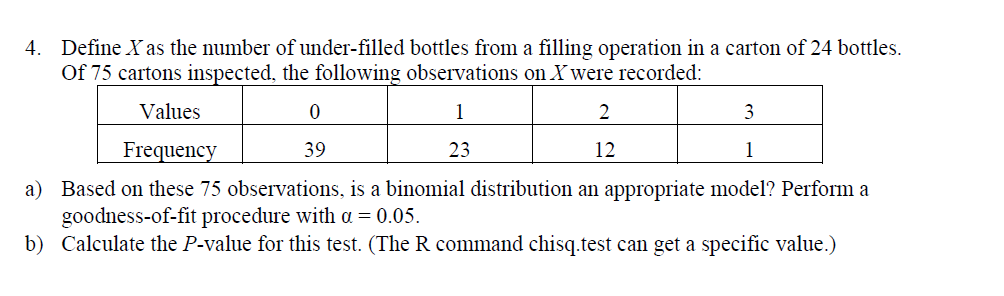# Define Yas the number of under-filled bottles from a filling operation in a carton of 24 bottles.Of 75 cartons inspected, the following observations on X were recorded4.ValuesFrequency39.312Based on these 75 observations, is a binomial distribution an appropriate model? Perform agoodness-of-fit procedure with a -0.05.a)b) Calculate the P-value for this test. (The R command chisq.test can get a specific value.)

Question
709 viewshelp_outlineImage TranscriptioncloseDefine Yas the number of under-filled bottles from a filling operation in a carton of 24 bottles. Of 75 cartons inspected, the following observations on X were recorded 4. Values Frequency 39 .3 12 Based on these 75 observations, is a binomial distribution an appropriate model? Perform a goodness-of-fit procedure with a -0.05. a) b) Calculate the P-value for this test. (The R command chisq.test can get a specific value.) fullscreen
check_circle

Step 1

Given information:

Denote the variable “number of under filled bottles from a filling operation of 24 bottles” as X.

The data represents the number of under filled bottles from a filling operation of 24 bottles among the 75 inspected cartons.

The specific probabilities for the variable “number of under filled bottles from a filling operation of 24 bottles” are given below:

Step 2

a.

Perform a goodness of fit procedure to test whether binomial distribution is an appropriate model for the data or not:

State the appropriate hypothesis.

The hypotheses are given below:

Null hypothesis:

H0 : The binomial distribution is an appropriate model for the data.

Alternative hypothesis:

H1 : The binomial distribution is not an appropriate model for the data.

Step 3

Obtain the test statistic value.

Here, MINITAB software is used to find the test statistic.

Software procedure:

Step by step procedure to obtain the test statistic using the MINITAB software:

• Choose Stat > Tables > Chi-square goodness of fit (One variable).
• In Observed counts enter the column of Frequency.
• In Category names enter the column of
• In Test select Specific proportions and enter the column of
• In Sample1 enter Trea...

### Want to see the full answer?

See Solution

#### Want to see this answer and more?

Solutions are written by subject experts who are available 24/7. Questions are typically answered within 1 hour.*

See Solution
*Response times may vary by subject and question.
Tagged in

### Other